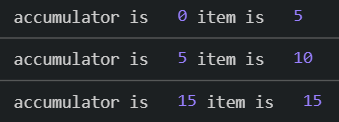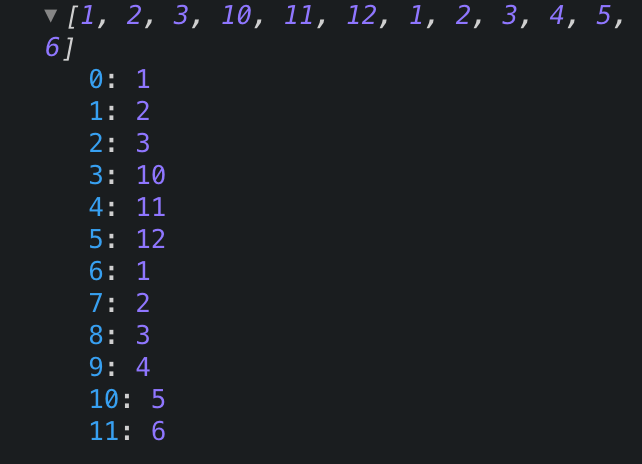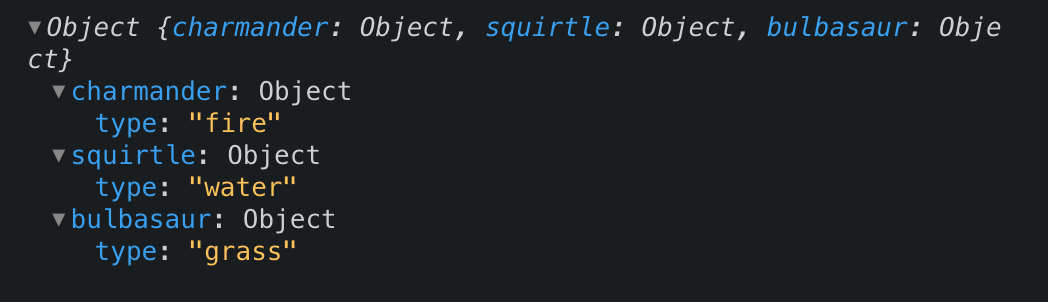## Finally Understand the JavaScript Reduce Method

November 07, 2019

Finally Understand the JavaScript Reduce Method

Reduce is a method that can be difficult to understand especially with all the vague explanations that can be found on the web. There are a lot of benefits to understanding `reduce` as it is often used in state management (think Redux).

The signature for the `reduce` array method in JavaScript is:

``````arr.reduce(callback, initialValue);
``````

## Terminology

Reduce comes with some terminology such as reducer & accumulator. The `accumulator` is the value that we end with and the `reducer` is what action we will perform in order to get to one value.

You must remember that a reducer will only return one value and one value only hence the name reduce.

Take the following classic example:

``````const value = 0; const numbers = [5, 10, 15]; for(let i = 0; i < numbers.length; i++) { value += numbers[i];
}
``````

The above will give us `30` (5 + 10 + 15). This works just fine, but we can do this with `reduce` instead which will save us from mutating our `value` variable.

The below code will also output `30`, but will not mutate our `value` variable (which we have now called `initialValue`)

``````/* this is our initial value i.e. the starting point/
const initialValue = 0; / numbers array /
const numbers = [5, 10, 15]; / reducer method that takes in the accumulator and next item /
const reducer = (accumulator, item) => { return accumulator + item;
}; / we give the reduce method our reducer function and our initial value */
const total = numbers.reduce(reducer, initialValue)
``````

The above code may look a little confusing, but under the hood there is no magic going on. Let’s add a `console.log` in our `reducer` method that will output the `accumulator` and the `item` arguments.

The following screenshot shows what’s logged to the console:So the first thing we notice is our method is called `3` times because there are `3` values in our array. Our accumulator begins at `0` which is our `initialValue` we passed to `reduce`. On each call to the function the `item` is added to the `accumulator`. The final call to the method has the `accumulator` value of `15` and `item` is `15`, `15 + 15` gives us `30` which is our final value. Remember the `reducer` method returns the `accumulator` plus the `item`.

So that is a simple example of how you would use `reduce`, now let’s dive into more a complicated example.

## Flattening an Array Using Reduce

Let’s say we have the following array:

``````const numArray = [1, 2, [3, 10, [11, 12]], [1, 2, [3, 4]], 5, 6];
``````

And let’s say for some crazy reason, JavaScript has removed the `.flat` method so we have to flatten this array ourselves.

So we’ll write a function to flatten any array no matter how deeply nested the arrays are:

``````function flattenArray(data) { // our initial value this time is a blank array const initialValue = []; // call reduce on our data return data.reduce((total, value) => { // if the value is an array then recursively call reduce // if the value is not an array then just concat our value return total.concat(Array.isArray(value) ? flattenArray(value) : value); }, initialValue);
}
``````

If we pass our `numArray` to this method and log the result we get the following:This is a great example on how we can make a very common operation quite simple.

Let’s go over one more example.

## Final Example - Changing an Object Structure

So with the new Pokemon game coming out, let’s pretend we have a server that sends us an array of Pokemon objects like so:

``````const pokemon = [ { name: "charmander", type: "fire" }, { name: "squirtle", type: "water" }, { name: "bulbasaur", type: "grass" }
]
``````

We want to change this object to look like:

``````const pokemonModified = { charmander: { type: "fire" }, squirtle: { type: "water" }, bulbasaur: { type: "grass" }
};
``````

To get to that desired output we do the following:

``````const getMapFromArray = data => data.reduce((acc, item) => { // add object key to our object i.e. charmander: { type: 'water' } acc[item.name] = { type: item.type }; return acc; }, {});
``````

If we call our method like so:

We get our desired output:You can check out the Codesandbox here.

## Conclusion

So that is it! Hope you’ve now learned how the reduce method works.